Courses

# Instrumentation Engineering - IN 2013 GATE Paper (Practice Test)

## 65 Questions MCQ Test GATE Past Year Papers for Practice (All Branches) | Instrumentation Engineering - IN 2013 GATE Paper (Practice Test)

Description
This mock test of Instrumentation Engineering - IN 2013 GATE Paper (Practice Test) for GATE helps you for every GATE entrance exam. This contains 65 Multiple Choice Questions for GATE Instrumentation Engineering - IN 2013 GATE Paper (Practice Test) (mcq) to study with solutions a complete question bank. The solved questions answers in this Instrumentation Engineering - IN 2013 GATE Paper (Practice Test) quiz give you a good mix of easy questions and tough questions. GATE students definitely take this Instrumentation Engineering - IN 2013 GATE Paper (Practice Test) exercise for a better result in the exam. You can find other Instrumentation Engineering - IN 2013 GATE Paper (Practice Test) extra questions, long questions & short questions for GATE on EduRev as well by searching above.
QUESTION: 1

Solution:
QUESTION: 2

Solution:
QUESTION: 3

### Choose the grammatically CORRECT sentence:

Solution:
QUESTION: 4

They were requested not to quarrel with others.

Which one of the following options is the closest in meaning to the word quarrel?

Solution:
QUESTION: 5

In the summer of 2012, in New Delhi, the mean temperature of Monday to Wednesday was 41ºC and of Tuesday to Thursday was 43ºC. If the temperature on Thursday was 15% higher than that of Monday, then the temperature in ºC on Thursday was

Solution:

Let the temperature of Monday be TM

Sum of temperatures of Tuesday and Wednesday = T and

Temperature of Thursday =TTh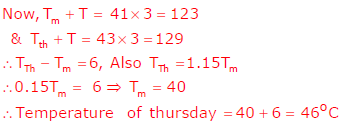QUESTION: 6

Q. No. 6 – 10 Carry Two Marks Each

Q. Find the sum to n terms of the series 10 + 84 + 734 + …

Solution: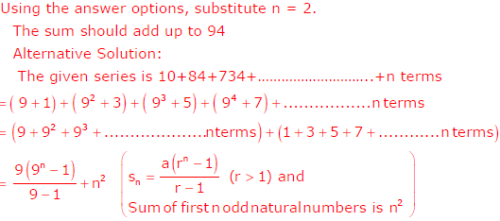QUESTION: 7

The set of values of p for which the roots of the equation 3x2 + 2x + p(p − 1) = 0 are of opposite sign is

Solution: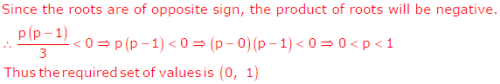QUESTION: 8

A car travels 8 km in the first quarter of an hour, 6 km in the second quarter and 16km in the third quarter. The average speed of the car in km per hour over the entire journey is

Solution: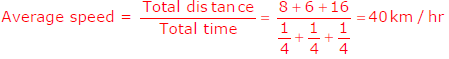QUESTION: 9

What is the chance that a leap year, selected at random, will contain 53 Sundays?

Solution: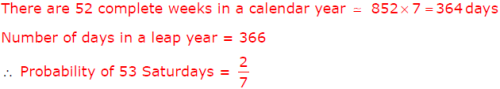QUESTION: 10

Statement: There were different streams of freedom movements in colonial India carried out by the moderates, liberals, radicals, socialists, and so on.

Which one of the following is the best inference from the above statement?

Solution:
QUESTION: 11

Q. No. 11 – 35 Carry One Mark Each

The dimension of the null space of the matrix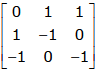is

Solution: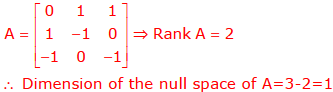QUESTION: 12

If the A- matrix of the state space model of a SISO linear time invariant system is rank deficient, the transfer function of the system must have

Solution:
QUESTION: 13

Two systems with impulse responses h1(t) and h2(t) are connected in cascade. Then the overall impulse response of the cascaded system is given by

Solution: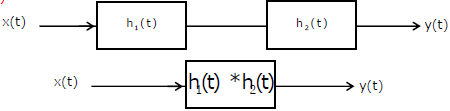QUESTION: 14

The complex function tanh (s) is analytic over a region of the imaginary axis of the complex s-plane if the following is TRUE everywhere in the region for all integers n

Solution: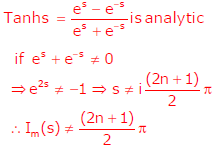QUESTION: 15

For a vector E, which one of the following statements is NOT TRUE?

Solution: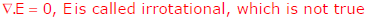QUESTION: 16

For a periodic signal v(t) = 30sin100t + 10cos300t  +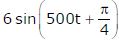, the fundamental frequency in rad/s is

Solution: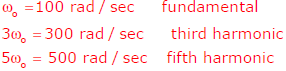QUESTION: 17

In the transistor circuit as shown below, the value of resistance RE in kW is approximately,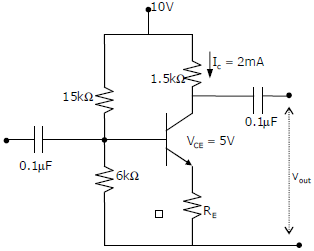Solution: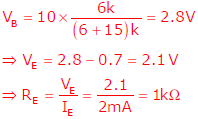QUESTION: 18

A source vs(t) = V cos100πt has an internal impedance of (4 + j3) ? . If a purely resistive load connected to this source has to extract the maximum power out of the source, its value in ? should be

Solution: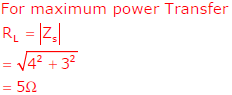QUESTION: 19

Which of the following statements is NOT TRUE for a continuous time causal and stable LTI system?

Solution:

For an LTI system to be stable and causal all poles or roots of characteristic equation must lie on LHS of s-plane i.e., left hand side of jω− axis

[Refer Laplace transform].

QUESTION: 20

The operational amplifier shown in the circuit below has a slew rate of 0.8V /μs . The input signal is 0.25sinωt . The maximum frequency of input in kHz for which there is no distortion in the output is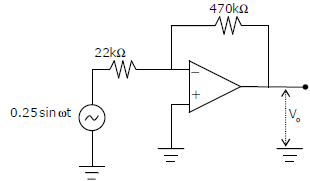Solution: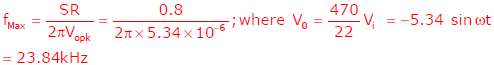QUESTION: 21

Assuming zero initial condition, the response y(t) of the system given below to a unit step input u(t) is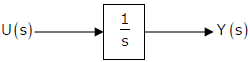Solution: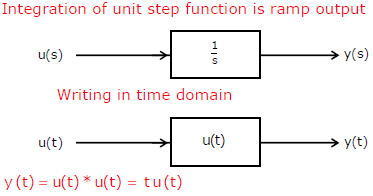QUESTION: 22

The transfer function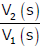of the circuit shown below is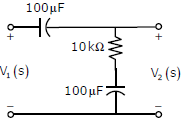Solution: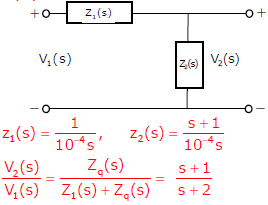QUESTION: 23

The type of partial differential equation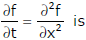Solution: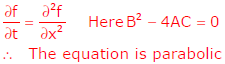QUESTION: 24

The discrete-time transfer function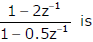Solution: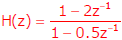For minimum phase system, all poles and zeros must lie inside the unit circle.

For stable system, all poles must be inside the unit circle

For the given system, Zero is at 2, pole is at 0.5

This system is stable but non-minimum phase

QUESTION: 25

Match the following biomedical instrumentation techniques with their application.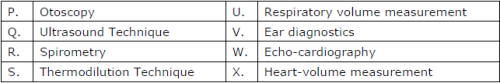Solution:
QUESTION: 26

A continuous random variable X has a probability density function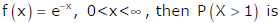Solution: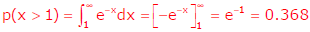QUESTION: 27

A band limited signal with a maximum frequency of 5 kHz is to be sampled. According to the sampling theorem, the sampling frequency in kHz which is not valid is

Solution:

Given: fm = 5kHz

According to sampling frequency

fs > 2fm ; fs > 10 KHz

So, only in option (a) it is less than 10KHz ie., (5KHz)

QUESTION: 28

A differential pressure transmitter of a flow meter using venture tube reads 2.5 ×105 Pa for a flow rate of 0.5 m3/s. The approximate flow rate in m3/s for a differential pressure of 0.9 ×105 Pa is

Solution: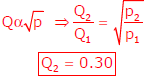QUESTION: 29

A bulb in a staircase has two switches, one switch being at the ground floor and the other one at the first floor. The bulb can be turned ON and also can be turned OFF by any one of the switches irrespective of the state of the other switch. The logic of switching of the bulb resembles

Solution: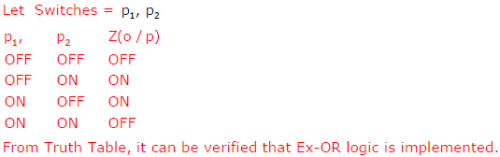QUESTION: 30

The impulse response of a system is h(t) = tu(t) . For an input u(t − 1) , the output is

Solution: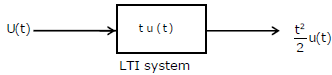For LTI system, if input gets delayed by one  unit, output will also get delayed by one unit.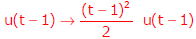QUESTION: 31

Consider a delta connection of resistors and its equivalent star connection as shown. If all elements of the delta connection are scaled by a factor k, k>0, the elements of the corresponding star equivalent will be scaled by a factor of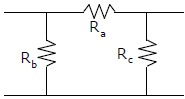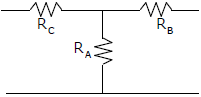Solution: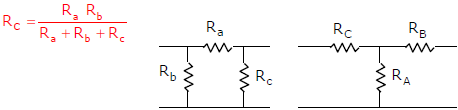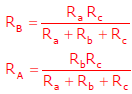Above expression shown that if Ra , Rb & Ris scaled by k, RA, RB & RC is scaled by k only.

QUESTION: 32

An accelerometer has input range of 0-10g, natural frequency 30Hz and mass 0.001kg. The range of the secondary displacement transducer in mm required to cover the input range is

Solution: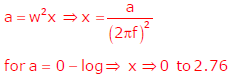QUESTION: 33

In the circuit shown below what is the output voltage V(out) in Volts if a silicon transistor Q and an ideal op-amp are used?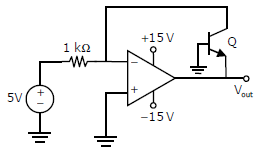Solution: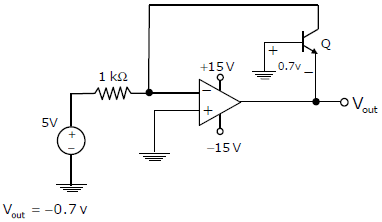QUESTION: 34

In the feedback network shown below, if the feedback factor k is increased, then the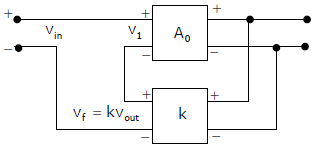Solution: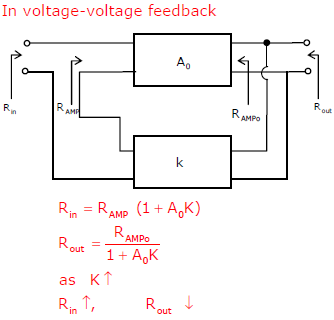QUESTION: 35

The Bode plot of a transfer function G(s) is shown in the figure below.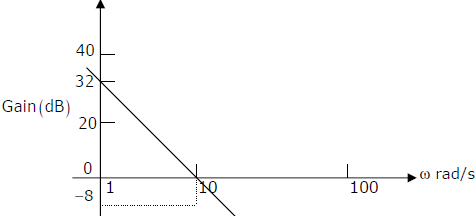The gain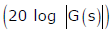is 32dB and -8dB at 1 rad/s and 10 rad/s respectively. The phase is negative for all ω. Then G(s) is

Solution:

Any two paints on same line segment of Bode plot satisfies the equation of straight line.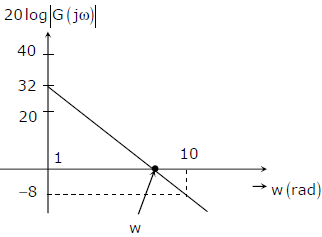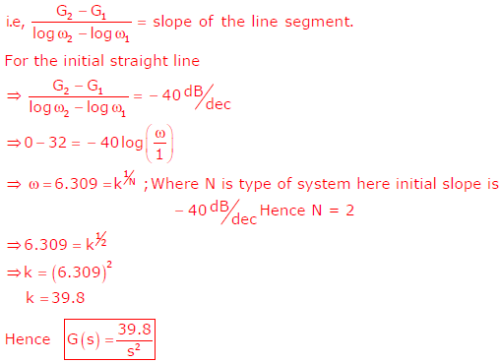QUESTION: 36

Q. No. 36 – 65 Carry Two Mark Each

Q. While numerically solving the differential equation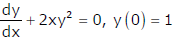using Euler's predictor– corrector (improved Euler – Cauchy) method with a step size of 0.2, the value of y after the first step is

Solution: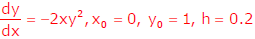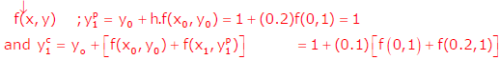= 0.96, is the value of y after first step, using Euler’s predictor – corrector method.

QUESTION: 37

One pair of eigen vectors corresponding to the two eigen values of the matrix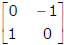is

Solution: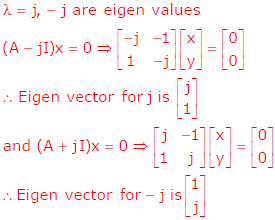QUESTION: 38

The digital circuit shown below uses two negative edge–triggered D–flip–flops. Assuming initial condition of Q1 and Q0 as zero, the output Q1Q0 of this circuit is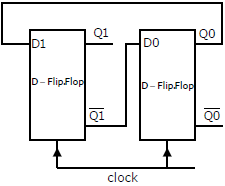Solution: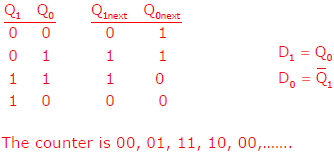QUESTION: 39

Considering the transformer to be ideal, the transmission parameter 'A' of the 2–port network shown in the figure below is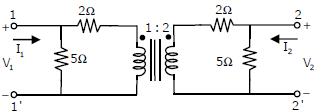Solution:
QUESTION: 40

The following arrangement consists of an ideal transformer and an attenuator, which attenuates by a factor of 0.8. An ac voltage VWX1 = 100V is applied across WX to get an open circuit voltage VYZ1  across YZ. Next, an ac voltage VYZ2 = 100V is applied across YZ to get an open circuit voltage VWX2 across WX. Then, VYZ1 / VWX1 , VWX2 VYZ2 are respectively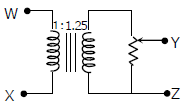Solution: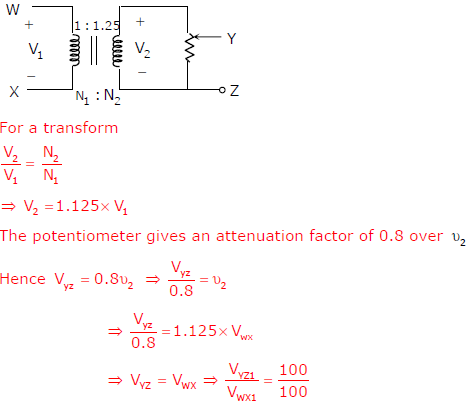Since potentiometer and transformer are bilateral elements. Hence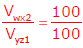QUESTION: 41

The open–loop transfer function of a dc motor is given as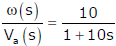. When connected in feedback as shown below, the approximate value of a Ka that will reduce the time constant of the closed loop system by one hundred times as compared to that of the open–loop system is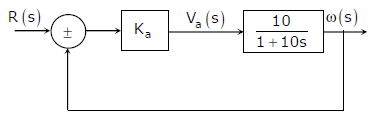Solution: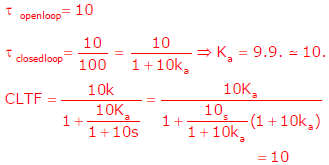QUESTION: 42

Two magnetically uncoupled inductive coils have Q factor q1 and q2 at the chosen operating frequency. Their respective resistances are R1 and R2 . When "connected in series, the effective Q factor of the series combination at the same operating frequency is

Solution: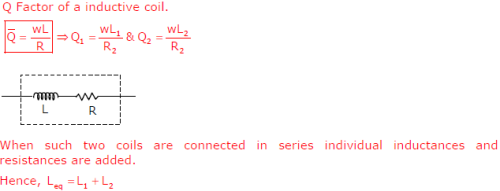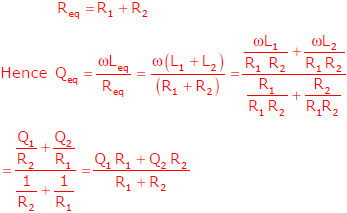QUESTION: 43

For the circuit shown below, the knee current of the ideal Zener diode is 10 mA. To maintain 5 V across RL , the minimum value of the load resistor RL in ? and the minimum power rating of the Zener diode in mW, respectively, are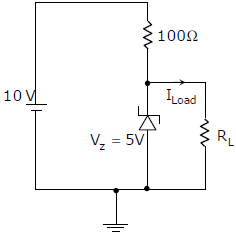Solution: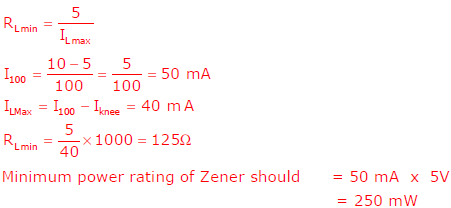QUESTION: 44

The impulse response of a continuous time system is given by h(t) = δ (t − 1) + δ (t − 3) . The value of the step response at t = 2 is

Solution: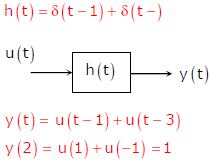QUESTION: 45

Signals from fifteen thermocouples are multiplexed and each one is sampled once per second with a16–bit  ADC. The digital samples are converted by a parallel to serial converter to generate a serial PCM signal. This PCM signal is frequency modulated with FSK modulator with 1200 Hz as 1 and 960 Hz as 0. The minimum
band allocation required for faithful reproduction of the signal by the FSK receiver without considering noise is

Solution:

Data rate from ADC is 16 x 15 bits/second=240 bits/second

The bandwidth required for transmitting 240 bits/second = 120 Hz. (Half of bit rate).

Now when each pulse representing ‘1’s and ‘0’ s get FSK modulator with 960 Hz and 1200

Base band signal before modulation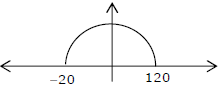The spectrum of signal after modulation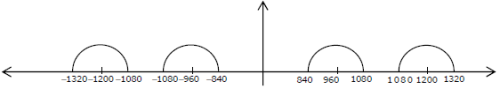Thus all frequency from 840 to 1320 is required for allocation

QUESTION: 46

Three capacitors C1 ,C2 and C3 whose values are 10μF,5μF and 2μF respectively, have breakdown voltages of 10V, 5V, and 2V respectively. For the interconnection shown below, the maximum safe voltage in Volts that can be applied across the combination, and the corresponding total charge in μC stored in the effective capacitance across the terminals are, respectively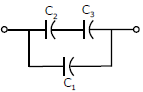Solution: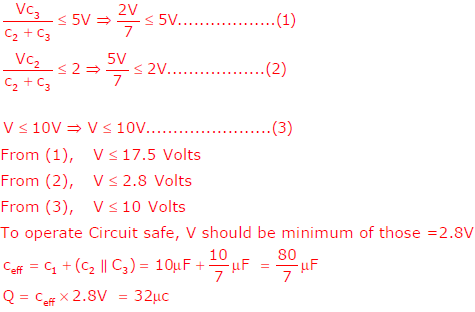QUESTION: 47

The maximum value of the solution y(t) of the differential equation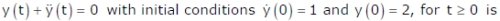Solution: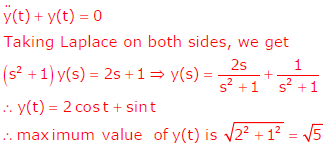QUESTION: 48

The Laplace Transform representation of the triangular pulse shown below is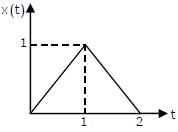Solution: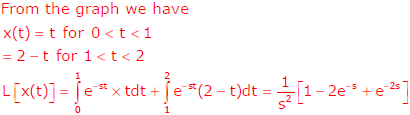QUESTION: 49

In the circuit shown below, if the source voltage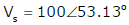Volts, then the Thevenin's equivalent voltage in Volts as seen by the load resistance RL is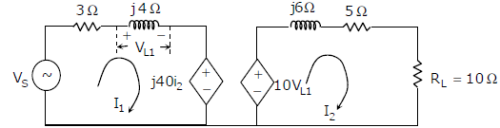Solution: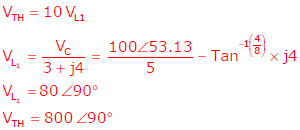QUESTION: 50

A signal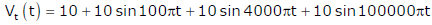is supplied to a filter circuit (shown below) made up of ideal op–amps. The least attenuated frequency component in the output will be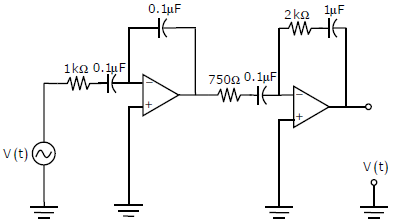Solution:

Least attenuated signal frequency is 0Hz

QUESTION: 51

The signal flow graph for a system is given below. The transfer function Y(s) / U(s) for this system is given as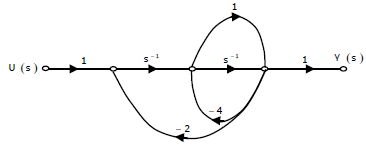Solution:

By using Mason’s gain formula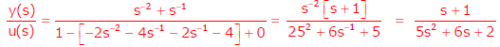QUESTION: 52

A voltage 1000 sin ωt Volts is applied across YZ. Assuming ideal diodes, the voltage measured across WX in Volts, is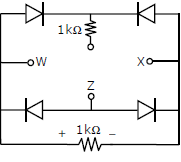Solution: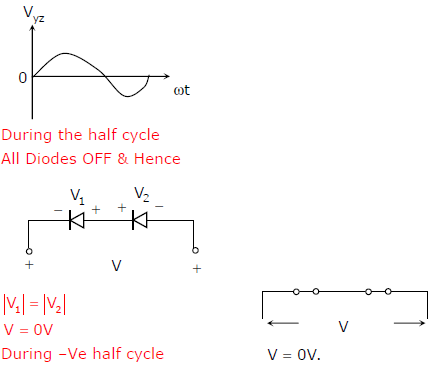QUESTION: 53

In the circuit shown below the op–amps are ideal. Then Vout in volts is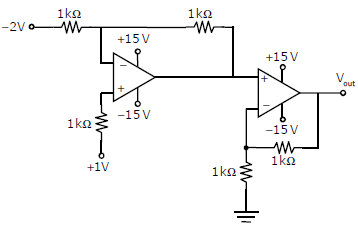Solution: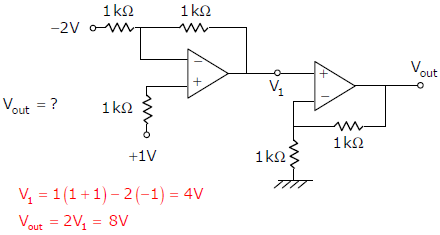QUESTION: 54

In the circuit shown below, Q1 has negligible collector–to–emitter saturation voltage and the diode drops negligible voltage across if under forward bias. If Vcc is + 5V , X and Y are digital signals with 0 V as logic 0 and Vcc as logic 1, then the Boolean expression for Z is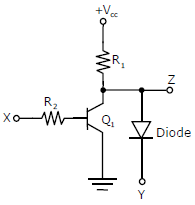Solution:

(B)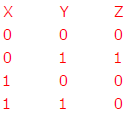QUESTION: 55

The circuit below incorporates a permanent magnet moving coil milli–ammeter of range 1 mA having a series resistance of 10k?. Assuming constant diode forward resistance of 50W a forward diode drop of 0.7 V and infinite reverse diode resistance for each diode, the reading of the meter in mA is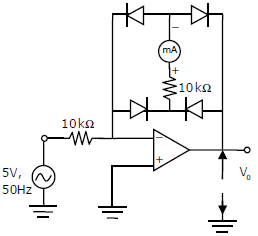Solution:
QUESTION: 56

Measurement of optical absorption of a solution is disturbed by the additional stray light falling at the photo-detector. For estimation of the error caused by stray light the following data could be obtained from controlled experiments.

Photo-detector output without solution and without stray light is 500 μW

Photo-detector output without solution and with stray light is 600 μW

Photo-detector output with solution and with stray light is 200 μW

The percent error in computing absorption coefficient due to stray light is

Solution:
QUESTION: 57

Two ammeters 1 A and 2 A measure the same current and provide readings Iand I2 respectively. The ammeter errors can be characterized as independent zero mean Gaussian random variables of standard deviations σ1 and σ2 respectively. The value of the current is computed as: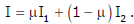. The value of V1 which gives the lowest standard deviation of I is

Solution:
QUESTION: 58

Common Data for Questions: 58 & 59

A tungsten wire used in a constant current hot wire anemometer has the following properties:

Resistance at 0ºC is 10?, Surface area is 10-4m2 . Linear temperature coefficient of resistance of the tungsten wire is 4.8 ×10−3/ºC , Convective heat transfer coefficient is 25.2W/m2 /ºC , flowing air temperature is 30ºC, wire current is 100mA, mass specific heat product is 2.5 x 105 J/ºC

Q. The thermal time constant of the hot wire under flowing air condition in ms is

Solution:
QUESTION: 59

Common Data for Questions: 58 & 59

A tungsten wire used in a constant current hot wire anemometer has the following properties:

Resistance at 0ºC is 10?, Surface area is 10-4m2 . Linear temperature coefficient of resistance of the tungsten wire is 4.8 ×10−3/ºC , Convective heat transfer coefficient is 25.2W/m2 /ºC , flowing air temperature is 30ºC, wire current is 100mA, mass specific heat product is 2.5 x 105 J/ºC

Q. At steady state, the resistance of the wire in ohms is

Solution:
QUESTION: 60

Common Data for Questions: 60 & 61

A piezo electric force sensor, connected by a cable to a voltage amplifier has the following parameters:

Crystal properties: Stiffness 106 N /m, Damping ratio 0.01, natural frequency 105 rad/s , Force-to-Charge sensitivity 10-9 C / N, Capacitance 10-9 F with its loss angle assumed negligible.

Cable properties : capacitance 2×10-9 F with its resistance assumed negligable Amplifier properties: Input impedance 1M?, Bandwidth 1MHz, Gain 3

Q. The maximum frequency of a force signal in Hz below the natural frequency within its useful midband range of measurement, for which the gain amplitude is less than 1.05 approximately is:

Solution:
QUESTION: 61

Common Data for Questions: 60 & 61

A piezo electric force sensor, connected by a cable to a voltage amplifier has the following parameters:

Crystal properties: Stiffness 106 N /m, Damping ratio 0.01, natural frequency 105 rad/s , Force-to-Charge sensitivity 10-9 C / N, Capacitance 10-9 F with its loss angle assumed negligible.

Cable properties : capacitance 2×10-9 F with its resistance assumed negligable Amplifier properties: Input impedance 1M?, Bandwidth 1MHz, Gain 3

Q. The minimum frequency of a force signal in Hz within its useful mid-band range of measurement for which the gain amplitude is more than 0.95 approximately is:

Solution:
QUESTION: 62

Linked Answer Questions: Q.62 to Q.65 Carry Two Marks Each

Statement for Linked Answer Questions: 62 & 63

Consider a plant with transfer function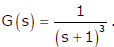. Let Ku and Tu be the ultimate gain and ultimate period corresponding to the frequency response based closed loop Ziegler-Nichols cycling method respectively. The Ziegler-Nichols tuning rule for a P-controller is given as: K = 0.5Ku

QThe values of Ku and Tu respectively are:

Solution:
QUESTION: 63

Linked Answer Questions: Q.62 to Q.65

Carry Two Marks Each Statement for Linked Answer Questions: 62 & 63

Consider a plant with transfer function G(s) = 1 / (s + 1)3. Let Ku and Tu be the ultimate gain and ultimate period corresponding to the frequency response based closed loop Ziegler-Nichols cycling method respectively. The Ziegler-Nichols tuning rule for a P-controller is given as: K = 0.5Ku

Q. The gain of the transfer function between the plant output and an additive load disturbance input of frequency 2π / Tin closed loop with a P-controller designed according to the Ziegler Nichols tuning rule as given above is

Solution:
QUESTION: 64

Statement for Linked Answer Questions: 64 & 65

A differential amplifier with signal terminals X, Y, Z is connected as shown in figure(a) below for CMRR measurement where the differential amplifier has an additional constant offset voltage in the output. The observations obtained are:

when Vi = 2V, Vo = 3mV and when Vi = 3V, Vo = 4mV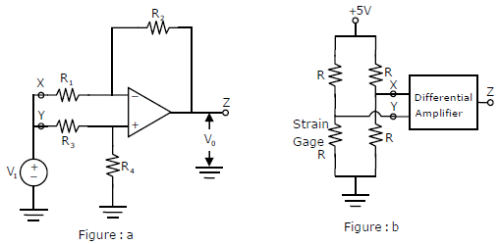Q. Assuming its differential gain to be 10 and the op-amp to be otherwise ideal, the CMRR is

Solution: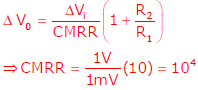QUESTION: 65

Statement for Linked Answer Questions: 64 & 65

A differential amplifier with signal terminals X, Y, Z is connected as shown in figure(a) below for CMRR measurement where the differential amplifier has an additional constant offset voltage in the output. The observations obtained are:

when Vi = 2V, Vo = 3mV and when Vi = 3V, Vo = 4mVQ. The differential amplifier is connected as shown in the figure(b) above to a single strain gage bridge. Let the strain gage resistance vary around its no load resistance R by ±1%. Assume the input impedance of the amplifier to be high compared to the equivalent source resistance of the bridge, and the common mode characteristic to be as obtained above. The output voltage in mV varies approximately from

Solution: